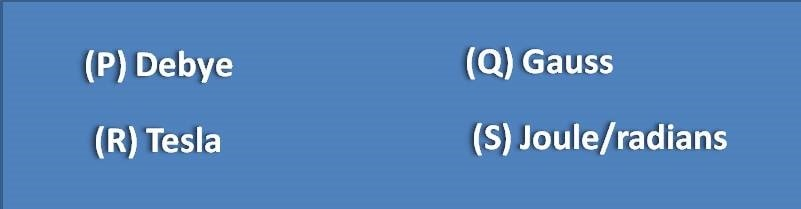# MCQ on ESR spectroscopy: Page-1

Which of the following will NOT show electron spin resonance (ESR)?

(B) Paramagnetic materials

(C) Transition metals

(D) Diamagnetic materials

Electron spin resonance is shown by compounds having unpaired electron. Electron has spin angular momentum as ½ and in case of paired electrons, the net spin will be zero as the electrons are paired with opposite spin. So ESR signal can’t be observed in diamagnetic materials where all the electrons are paired with opposite spin.

Units for magnetic field strength are(A) R and S

(B) P and Q

(C) Q and R

(D) P and S

Both Tesla and Gauss are the units for magnetic field strength. Tesla is the SI unit whereas Gauss is CGS unit.

Which of the following electromagnetic radiation is used in ESR?

(D) Microwaves

ESR spectra of a free radical were observed as shown below. The hyperfine splitting observed in the spectra may be due to a single nuclei of(A) 1H

(B) 12C

(C) 19F

(D) 14N

Spectra shown above contains 3 peak splitting of electron resonance line. It is possible by either with a nuclei I=1 or with three equivalent nuclei with I=½ . Among the options given, nitrogen has I=1, so it brings 3 splitting of resonance line.

For an unbound electron, value of Lande’ factor (g-factor) will be equal to

(A) 1.0098

(B) 2.0023

(C) 3.0015

(D) 6.0621